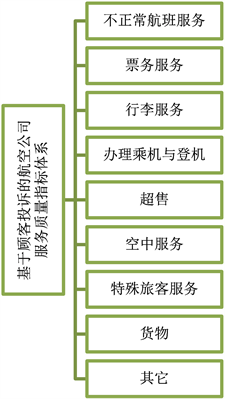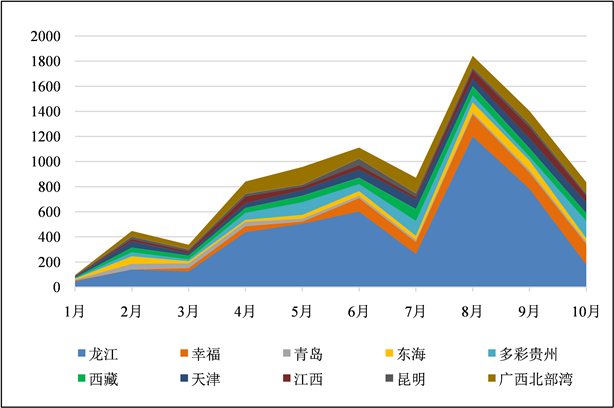﻿ 一种基于增益水平激励的航空公司服务质量动态综合评价模型

# 一种基于增益水平激励的航空公司服务质量动态综合评价模型A Dynamic Comprehensive Evaluation Model of Airline Service Quality Based on Gain Level Excitation

Abstract: Aiming at the change of complaint rate of different airlines in continuous time, according to the difference of static service quality growth rate at different time points, a dynamic comprehensive evaluation model of airline service quality based on gain level incentive was proposed. Firstly, the advantages and disadvantages of all the airlines’ gain level is determined, according to which the advantages and disadvantages of each airline incentive points at different time points are calculated. Secondly, the advantages and disadvantages of incentive factors are introduced to calculate the “reward” and “punishment” of different airlines relative to the advantages and disadvantages of incentive points, so as to obtain the final dynamic comprehensive evaluation value. Finally, taking the statistical data of Civil Aviation Administration from January to September in 2020 as an example, the dynamic service quality of ten companies such as Tianjin Airlines is analyzed, and the influence of different incentive factors on their ranking is analyzed, so as to verify the effectiveness of the model.

1. 引言

“十四五”时期，全行业将进入多领域民航强国建设新征程。人民群众航空服务需求将进一步提升，航空公司在服务质量方面的工作也面临更高的挑战。目前，国内航空公司的服务质量和水平参次不齐，相对较低的服务水平将会造成旅客的流失，进而减少航空公司的经济效益。因此，如何准确评价和提升服务质量，在日趋激烈的航空市场竞争中将是航空企业面临的重要问题之一  。现有航空公司服务质量评价的方法没有统一、规范的标准，较为常见的方法主要有二：一是通过问卷调查主要收集顾客的意见和建议，定性评价，缺乏科学系统的理论体系，也无法为改进航空服务质量指明前进的方向 ；二是建立指标体系，系统地进行工作质量综合打分，基于数学评价模型，量化每个指标的数值变化如何影响服务水平的临界 。

2. 基于顾客投诉的航空公司服务质量指标体系Figure 1. Index system

3. 航空公司服务质量的动态综合评价模型

${\rho }^{\mathrm{max}}=\underset{i}{\mathrm{max}}\frac{1}{T-1}{\sum }_{k=1}^{T-1}\left({y}_{i}\left({t}_{k+1}\right)-{y}_{i}\left({t}_{k}\right)\right)$

${\rho }^{\mathrm{min}}=\underset{i}{\mathrm{min}}\frac{1}{T-1}{\sum }_{k=1}^{T-1}\left({y}_{i}\left({t}_{k+1}\right)-{y}_{i}\left({t}_{k}\right)\right)$

$\stackrel{¯}{\rho }=\underset{i}{\mathrm{min}}\frac{1}{n\left(T-1\right)}{\sum }_{i=1}^{n}{\sum }_{k=1}^{T-1}\left({y}_{i}\left({t}_{k+1}\right)-{y}_{i}\left({t}_{k}\right)\right)$

${\rho }^{+}=\stackrel{¯}{\rho }+{k}^{+}\left({\rho }^{\mathrm{max}}-\stackrel{¯}{\rho }\right)$

${\rho }^{-}=\stackrel{¯}{\rho }-{k}^{-}\left(\stackrel{¯}{\rho }-{\rho }^{\mathrm{min}}\right)$

${\rho }^{+}={y}_{i}^{+}\left({t}_{k}\right)-{y}_{i}\left({t}_{k-1}\right)$

${\rho }^{-}=-{y}_{i}^{-}\left({t}_{k}\right)+{y}_{i}\left({t}_{k-1}\right)$

${v}_{i}^{+}\left({t}_{k}\right)=\left\{\begin{array}{ll}{y}_{i}^{+}\left({t}_{k}\right)-{y}_{i}\left({t}_{k}\right)\hfill & {y}_{i}^{+}\left({t}_{k}\right)>{y}_{i}\left({t}_{k}\right)\hfill \\ 0,\hfill & 其它\hfill \end{array}$

${v}_{i}^{-}\left({t}_{k}\right)=\left\{\begin{array}{ll}{y}_{i}\left({t}_{k}\right)-{y}_{i}^{-}\left({t}_{k}\right)\hfill & {y}_{i}\left({t}_{k}\right)>{y}_{i}^{-}\left({t}_{k}\right)\hfill \\ 0,\hfill & 其它\hfill \end{array}$

${z}_{i}\left({t}_{k}\right)={y}_{i}\left({t}_{k}\right)+{h}^{+}{v}_{i}^{+}\left({t}_{k}\right)-{h}^{-}{v}_{i}^{-}\left( t k \right)$

${z}_{i}={\sum }_{k=1}^{T}{\tau }_{k}{z}_{i}\left( t k \right)$

4. 应用实例Table 1. Airline service quality data from January to October 2019Figure 2. Changes in airline service quality rankings from January to October 2019Table 2. v i + ( t k )Table 3. v i − ( t k )Table 4. The service quality ranking of airlines in different months and the comprehensive ranking of the whole year

5. 结论

1) 部分航空公司之间服务质量排名稳定，但排名在不同月份会发生波动。因此，需要综合某段时间内的排名波动情况，综合动态评价航空公司的服务质量水平。

2) 由于r = 0.003，小于1，表示劣激励总量占优，即为了提升航空公司的服务质量水平，加大惩罚部分对排名的影响。

NOTES

*通讯作者。

 沙永全. 航空公司服务质量问题的成因分析[J]. 世界标准化与质量管理, 2005(7): 35-36.

 吴晖. 航空公司服务质量旅客满意度研究[J]. 现代商业, 2008(10): 175-176.

 赵凤彩, 吴婧. 国内外航空公司服务质量的对比分析[J]. 中国民用航空, 2009(9): 57-59.

 赵礼强, 潘杰, 唐金环. 民航企业绩效提升的多重路径研究——基于模糊集定性比较分析[J]. 沈阳航空航天大学学报, 2020(5): 63-71.

 付娉娉, 吴冲. 基于FAHP方法的国内航空公司竞争力评价研究[J]. 苏州大学学报(哲学社会科学版), 2011(2): 131-136.

 章玲, 张露平, 周鹏. 航空公司服务质量评价——基于非可加直觉模糊VIKOR方法[J]. 技术经济与管理研究, 2014(4): 8-14.

 李玲. 航空公司竞争力的灰色综合评价[J]. 时代金融, 2015(24): 184+190.

 杨超, 霍连才, 张红智. 基于灰色关联分析的航空公司旅客满意度评价研究[J]. 企业研究, 2014(4): 5-6.

 白钊, 杨琳. 基于DEA模型的国内航空公司服务绩效评价方法[J]. 航空计算技术, 2020(1): 9-12.

 张君, 胡荣, 李天睿. 基于三阶因子结构的航空公司服务质量评估模型研究[J]. 华东交通大学学报, 2014(5): 37-43.

 田利军, 谢箷. 基于因子分析法的航空公司核心竞争力评价[J]. 财会月刊, 2013(18): 72-75.

 马赞福, 郭亚军, 张发明, 潘玉厚. 一种基于增益水平激励的动态综合评价方法[J]. 系统工程学报, 2009, 24(2): 243-247.

 张发明. 基于双重激励模型的动态综合评价方法及应用[J]. 系统工程学报, 2013, 28(2): 248-255.

Top